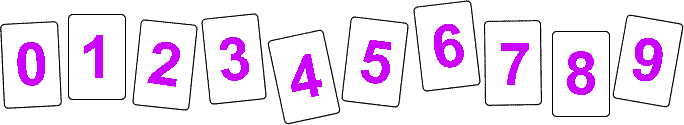#### You may also like### Prompt Cards

These two group activities use mathematical reasoning - one is numerical, one geometric.### Round and Round the Circle

What happens if you join every second point on this circle? How about every third point? Try with different steps and see if you can predict what will happen.### Tom's Number

Work out Tom's number from the answers he gives his friend. He will only answer 'yes' or 'no'.

# Penta Primes

##### Age 7 to 11 Challenge Level:

Here are ten cards numbered 0 to 9:Using all ten cards, rearrange them to make five prime numbers.

Can you find a way of doing it with five two-digit numbers?

How about using one one-digit number, one three-digit number and three two-digit numbers? ...

This problem is based on an idea from Mathematical Challenges for Able Pupils Key Stages 1 and 2, published by DfES.